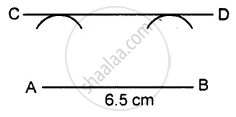# Draw a Straight Line Ab = 6.5 Cm. Draw Another Line Which is Parallel to Ab at a Distance of 2.8 Cm from It. - Mathematics

Sum

Draw a straight line AB = 6.5 cm. Draw another line which is parallel to AB at a distance of 2.8 cm from it.

#### Solution

Steps of construction :1. Draw a straight line AB = 6.5 cm
2. Taking point A as a centre, draw an arc of radius 2.8 cm.
3. Taking B as centre, drawn another arc of radius 2.8 cm.
4. Draw a line CD that touches the two arcs drawn.
Thus CD is the required parallel line.
Concept: Construction of an Angle - Construction of Parallel Lines
Is there an error in this question or solution?

#### APPEARS IN

Selina Concise Mathematics Class 8 ICSE
Chapter 18 Constructions
Exercise 18 (C) | Q 3 | Page 205